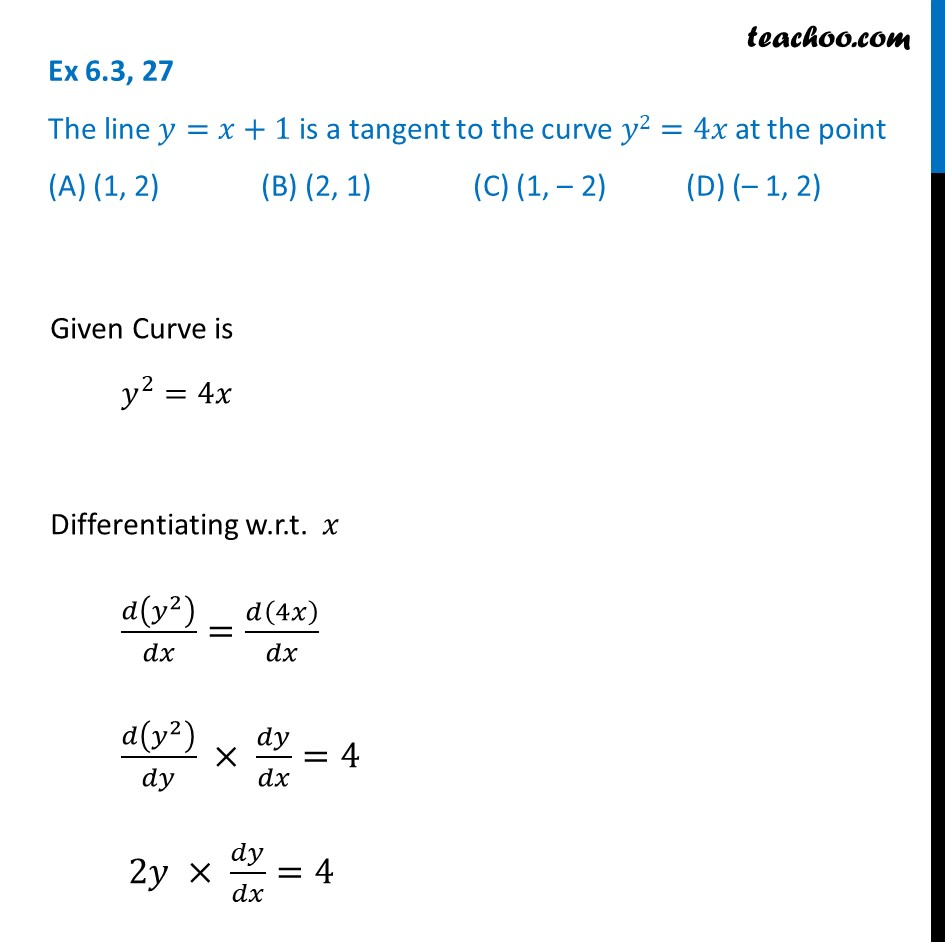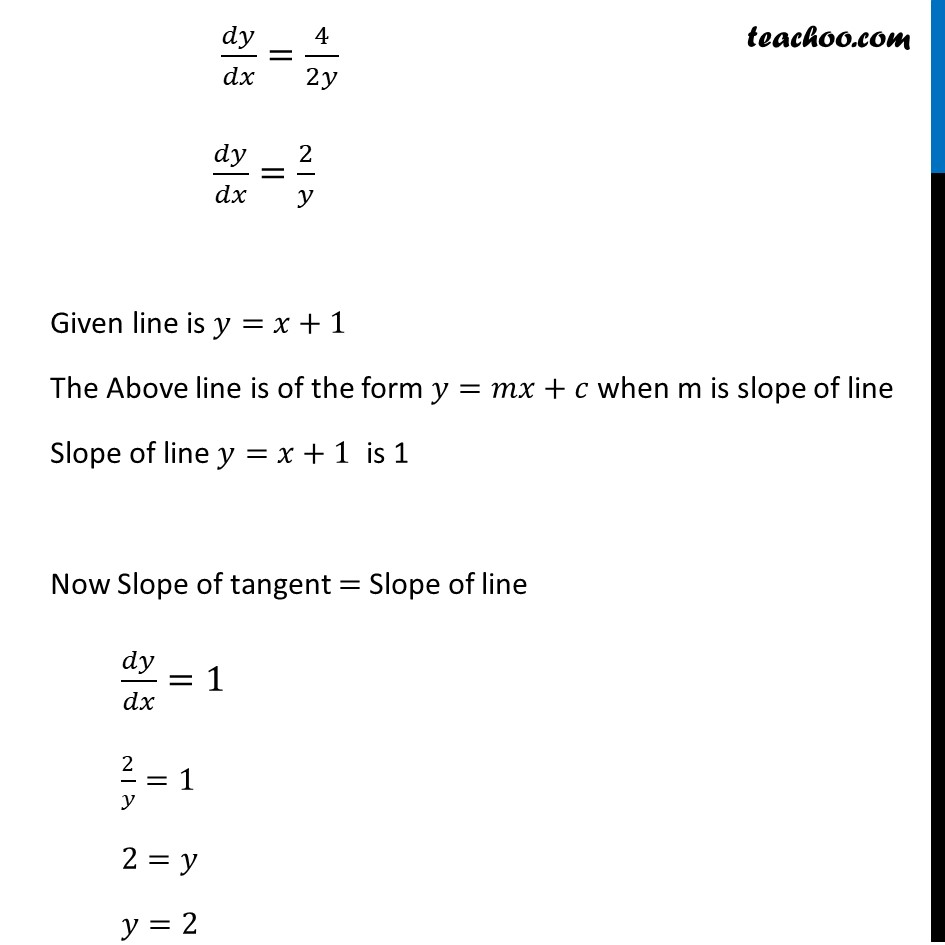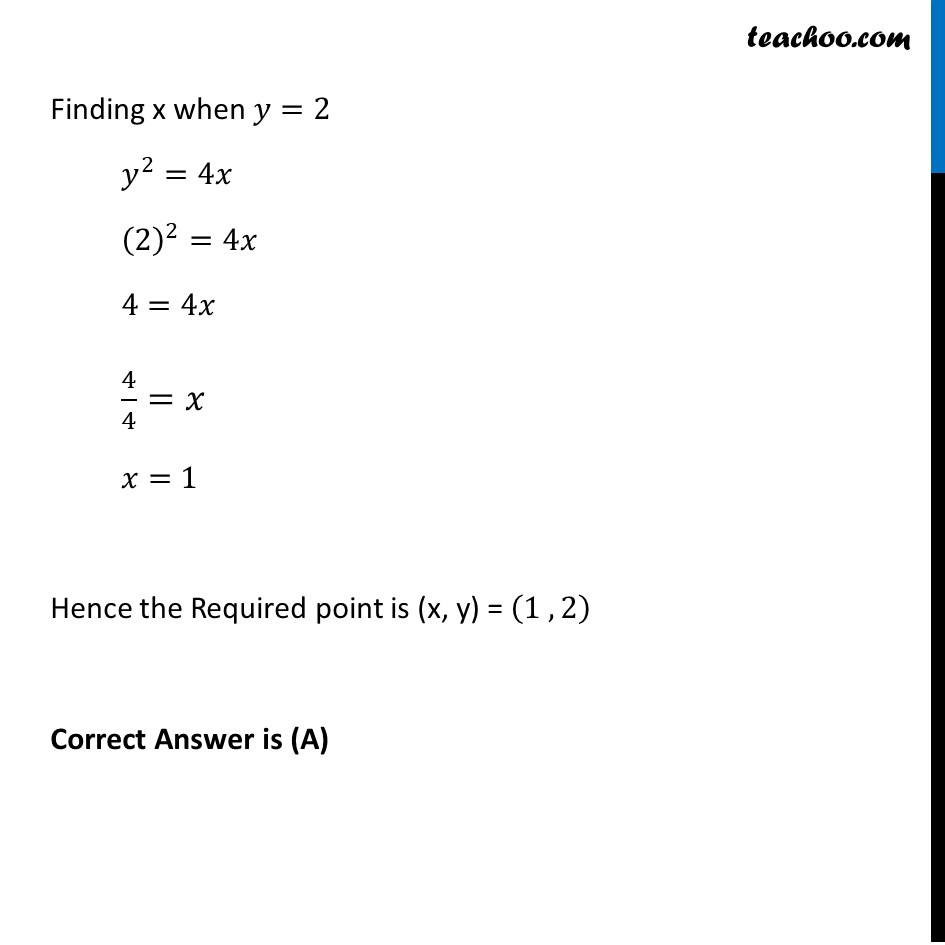Tangents and Normals (using Differentiation)

Chapter 6 Class 12 Application of Derivatives
Serial order wiseLearn in your speed, with individual attention - Teachoo Maths 1-on-1 Class

### Transcript

Question 27 The line 𝑦=𝑥+1 is a tangent to the curve 𝑦2=4𝑥 at the point (A) (1, 2) (B) (2, 1) (C) (1, – 2) (D) (– 1, 2)Given Curve is 𝑦^2=4𝑥 Differentiating w.r.t. 𝑥 𝑑(𝑦^2 )/𝑑𝑥=𝑑(4𝑥)/𝑑𝑥 𝑑(𝑦^2 )/𝑑𝑦 × 𝑑𝑦/𝑑𝑥=4 2𝑦 × 𝑑𝑦/𝑑𝑥=4 𝑑𝑦/𝑑𝑥=4/2𝑦 𝑑𝑦/𝑑𝑥=2/𝑦 Given line is 𝑦=𝑥+1 The Above line is of the form 𝑦=𝑚𝑥+𝑐 when m is slope of line Slope of line 𝑦=𝑥+1 is 1 Now Slope of tangent = Slope of line 𝑑𝑦/𝑑𝑥=1 2/𝑦=1 2=𝑦 𝑦=2 Finding x when 𝑦=2 𝑦^2=4𝑥 (2)^2=4𝑥 4=4𝑥 4/4=𝑥 𝑥=1 Hence the Required point is (x, y) = (1 , 2) Correct Answer is (A)